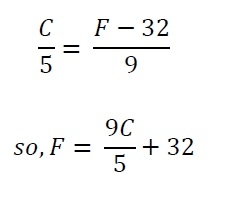# C++ program to convert Fahrenheit to Celsius

C++Server Side ProgrammingProgramming

In this program we will see how to convert Celsius to Fahrenheit using C++. As we know the formula is simple.## Algorithm

Begin
Take the Celsius temperature in C
calculate F = (9C/5)+32
return F
End

## Example Code

#include<iostream>
using namespace std;
main() {
float f, c;
cout << "Enter temperature in Celsius: ";
cin >> c;
f = (9.0*c/5.0)+32;
cout << "Equivalent Fahrenheit temperature is: " << f;
}

## Output

Enter temperature in Celsius: 37
Equivalent Fahrenheit temperature is: 98.6